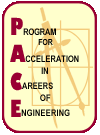Classes

PACE - Monmouth students take one math and one engineering class each year. In general, students take a math class that matches the math they take at their high school. Engineering classes follow a set sequence from ninth through twelfth grades.

Math

PACE math classes are meant to reinforce the math taken in school. When possible, students are assigned a math class that matches their high school math class. PACE offers the following five math classes:

See the web page for each class to get additional information.

Engineering

Engineering classes expose student to a few areas of engineering and teach basic concepts. All the engineering classes are hands-on and project oriented.

Students that enter PACE in the ninth grade take each of the four engineering classes in the listed order:

Students that enter the program in tenth grade or later may start with an engineering class other than Intro, or skip one of the other classes in later years.

See the web page for each class to get additional information.

Electives

New in 2008-09 is an elective PACE class.